## Linear Stability

Consider the general system of two first-order Ordinary Differential Equations(1)(2)

Let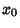and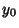denote Fixed Points with, so(3)(4)

Then expand aboutso(5)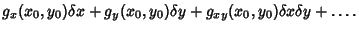(6)

To first-order, this gives(7)

where the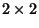Matrix is called the Stability Matrix.

In general, given an-D Map, letbe a Fixed Point, so that(8)(9)

so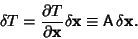(10)

The map can be transformed into the principal axis frame by finding the Eigenvectors and Eigenvalues of the Matrix A(11)

so the Determinant(12)

The mapping is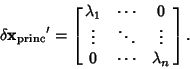(13)

When iterated a large number of times,(14)

only iffor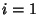, ...,butif any. Analysis of the Eigenvalues (and Eigenvectors) of A therefore characterizes the type of Fixed Point. The condition for stability isfor, ...,.

Tabor, M. Linear Stability Analysis.'' §1.4 in Chaos and Integrability in Nonlinear Dynamics: An Introduction. New York: Wiley, pp. 20-31, 1989.